# 1D - Heat equation

Finite differences applied to the solution of a 1D heat equation PDE.
Consider the following 1D heat problem where the temperature function depends on space and time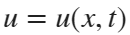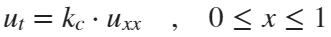with initial condition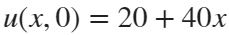and boundary conditions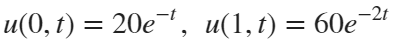.
Solve this problem for the parameter values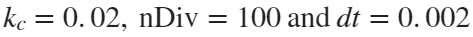and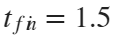The finite differences equation are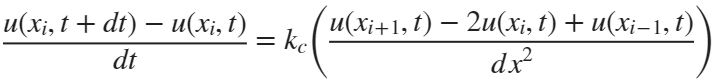if we define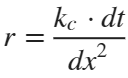then it can written in terms of components as: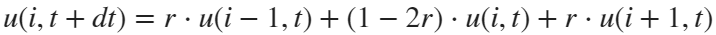It is also equivalent to write: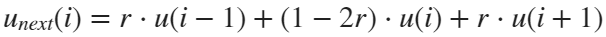kc = 0.02; %conductivity coefficient
xini = 0;
xfin = 1;
L = xfin-xini; %bar length
nDiv = 100; %number of spatial subdivisions
dx = L/nDiv; % spatial step
tini=0;
tfin = 1.5; %final time for the simulation
dt = 0.002; %time step
r = kc*dt/dx^2; %stability parameter (r<=0.5)
if (r > 0.5)
disp('unstable simulation');
return;
end
x = xini:dx:xfin;
u = 20+40*x';
unext = u;
for t = dt:dt:tfin
for i = 2:mx
unext(i) = r*u(i-1)+(1-2*r)*u(i)+r*u(i+1);
end
% boundary conditions
unext(1) = 20*exp(-t);
unext(end) = 60*exp(-2*t);
% plot solution
plot(x, unext);
axis([-0.1,1.1, 0, 61]);
xlabel('x value');
ylabel('temperature')
text(0.2,50,['time = ' num2str(t)]);
grid;
drawnow;
u = unext;
end